# 1433 and Level 2

There is only one way to arrange the numbers from 1 to 10 in both the first column and the top row to make this puzzle function like a multiplication table. Can you find that one way?Print the puzzles or type the solution in this excel file:  10 Factors 1432-1442

The puzzle number is 1433. Here are some facts about that number:

• 1433 is a prime number.
• Prime factorization: 1433 is prime.
• 1433 has no exponents greater than 1 in its prime factorization, so √1433 cannot be simplified.
• The exponent in the prime factorization is 1. Adding one to that exponent we get (1 + 1) = 2. Therefore 1433 has exactly 2 factors.
• The factors of 1433 are outlined with their factor pair partners in the graphic below.

How do we know that 1433 is a prime number? If 1433 were not a prime number, then it would be divisible by at least one prime number less than or equal to √1433. Since 1433 cannot be divided evenly by 2, 3, 5, 7, 11, 13, 17, 19, 23, 29 31, or 37, we know that 1433 is a prime number.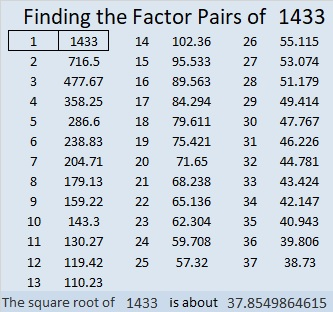1433 is the sum of two squares:
37² + 8² = 1433

1433 is the hypotenuse of a Pythagorean triple:
592-1305-1433 calculated from 2(37)(8), 37² – 8², 37² + 8²

Here’s another way we know that 1433 is a prime number: Since its last two digits divided by 4 leave a remainder of 1, and 37² + 8² = 1433 with 37 and 8 having no common prime factors, 1433 will be prime unless it is divisible by a prime number Pythagorean triple hypotenuse less than or equal to √1433. Since 1433 is not divisible by 5, 13, 17, 29, or 37, we know that 1433 is a prime number.

# 1420 Eight-legged Creature

When you look at today’s puzzle, you might see a snowflake or perhaps an eight-legged creature. I hope you will also see lots of common factors that will help you solve this quick puzzle.Print the puzzles or type the solution in this excel file: 12 Factors 1419-1429

Here are some facts about the puzzle number, 1420:

• 1420 is a composite number.
• Prime factorization: 1420 = 2 × 2 × 5 × 71, which can be written 1420 = 2² × 5 × 71
• 1420 has at least one exponent greater than 1 in its prime factorization so √1420 can be simplified. Taking the factor pair from the factor pair table below with the largest square number factor, we get √1420 = (√4)(√355) = 2√355
• The exponents in the prime factorization are 2, 1, and 1. Adding one to each exponent and multiplying we get (2 + 1)(1 + 1)(1 + 1) = 3 × 2 × 2 = 12. Therefore 1420 has exactly 12 factors.
• The factors of 1420 are outlined with their factor pair partners in the graphic below.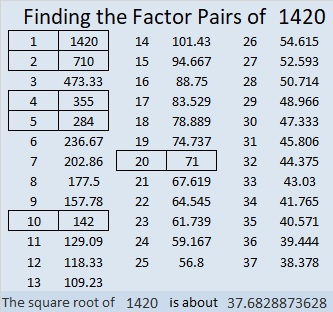1420 is the hypotenuse of a Pythagorean triple:
852-1136-1420 which is (3-4-5) times 284.

# 1411 and Level 2

Four of the fourteen clues, 18, 24, 16, and 40, appear twice in this puzzle, but do they lead you to the same factors? Where do the factors from 1 to 10 belong that will make this puzzle function like a multiplication table?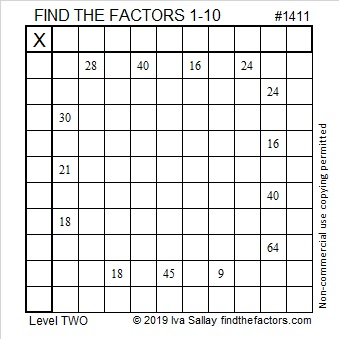Print the puzzles or type the solution in this excel file: 10 Factors 1410-1418

Now I’ll write a little bit about the puzzle number, 1411:

• 1411 is a composite number.
• Prime factorization: 1411 = 17 × 83
• 1411 has no exponents greater than 1 in its prime factorization, so √1411 cannot be simplified.
• The exponents in the prime factorization are 1, and 1. Adding one to each exponent and multiplying we get (1 + 1)(1 + 1) = 2 × 2 = 4. Therefore 1411 has exactly 4 factors.
• The factors of 1411 are outlined with their factor pair partners in the graphic below.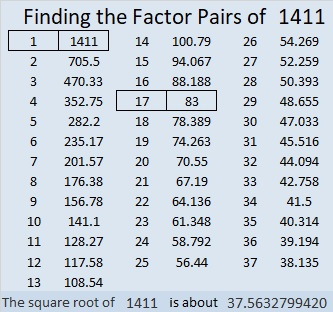1411 is the hypotenuse of a Pythagorean triple:
664-1245-1411 which is (8-15-17) times 83.

# 1394 and Level 2

The factors and most of the products are missing from this multiplication table, and the ones that are there aren’t in there usual places. Can you figure out where everything goes?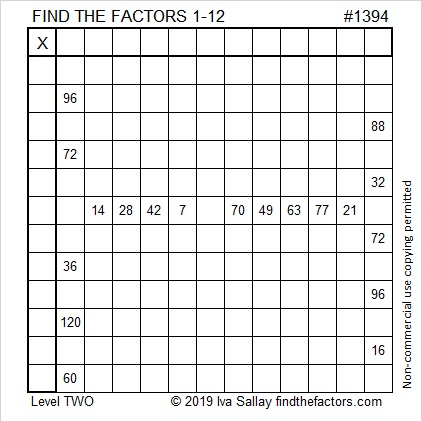Print the puzzles or type the solution in this excel file: 12 Factors 1389-1403

Now I’ll write a little bit about the puzzle number, 1394:

• 1394 is a composite number.
• Prime factorization: 1394 = 2 × 17 × 41
• 1394 has no exponents greater than 1 in its prime factorization, so √1394 cannot be simplified.
• The exponents in the prime factorization are 1, 1, and 1. Adding one to each exponent and multiplying we get (1 + 1)(1 + 1)(1 + 1) = 2 × 2 × 2 = 8. Therefore 1394 has exactly 8 factors.
• The factors of 1394 are outlined with their factor pair partners in the graphic below.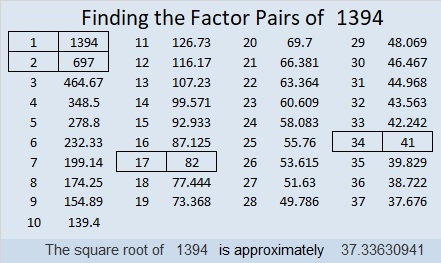1394 is the hypotenuse of FOUR Pythagorean triples:
306-1360-1394 which is (9-40-41) times 34
370-1344-1394 which is 2 times (185-672-697)
656-1230-1394 which is (8-15-17) times 82
910-1056-1394 which is 2 times (455-528-697)

# 1374 and Level 2

Can you find the factors that will turn this puzzle into a multiplication table whose products are simply not in the usual order?Print the puzzles or type the solution in this excel file: 10 Factors 1373-1388

Here are a few facts about the puzzle number, 1374:

• 1374 is a composite number.
• Prime factorization: 1374 = 2 × 3 × 229
• 1374 has no exponents greater than 1 in its prime factorization, so √1374 cannot be simplified.
• The exponents in the prime factorization are 1, 1, and 1. Adding one to each exponent and multiplying we get (1 + 1)(1 + 1)(1 + 1) = 2 × 2 × 2 = 8. Therefore 1374 has exactly 8 factors.
• The factors of 1374 are outlined with their factor pair partners in the graphic below.1374 is the hypotenuse of a Pythagorean triple:
360-1326-1374 which is 6 times (60-221-229)

# 1358 and Level 2

If you are familiar with a basic 12 × 12 multiplication table, then you can solve this puzzle. The clues aren’t in the same order as they are in the table, but that only makes it a little more challenging.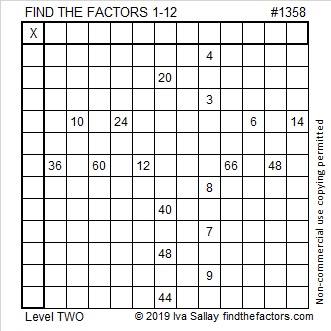Print the puzzles or type the solution in this excel file: 12 Factors 1357-1365

Here’s a little bit about the number 1358:

• 1358 is a composite number.
• Prime factorization: 1358 = 2 × 7 × 97
• 1358 has no exponents greater than 1 in its prime factorization, so √1358 cannot be simplified.
• The exponents in the prime factorization are 1, 1, and 1. Adding one to each exponent and multiplying we get (1 + 1)(1 + 1)(1 + 1) = 2 × 2 × 2 = 8. Therefore 1358 has exactly 8 factors.
• The factors of 1358 are outlined with their factor pair partners in the graphic below.910-1008-1358 which is 14 times (65-72-97)

Stetson.edu informs us that 1358!!!! + 1 is a prime number.

# 1351 and a Level 2 Smiley Face

You will smile a great big smile once you solve this puzzle!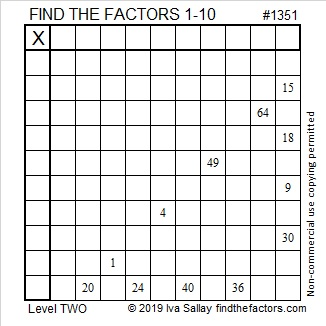Print the puzzles or type the solution in this excel file: 10 Factors 1347-1356

If you want a little help solving the puzzle, I explain how to do it in this video:

Here are some facts about the puzzle number 1351:

• 1351 is a composite number.
• Prime factorization: 1351 = 7 × 193
• 1351 has no exponents greater than 1 in its prime factorization, so √1351 cannot be simplified.
• The exponents in the prime factorization are 1, and 1. Adding one to each exponent and multiplying we get (1 + 1)(1 + 1) = 2 × 2 = 4. Therefore 1351 has exactly 4 factors.
• The factors of 1351 are outlined with their factor pair partners in the graphic below.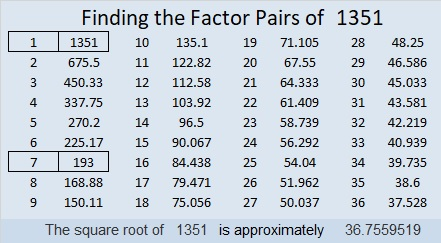1351 is the hypotenuse of a Pythagorean triple:
665-1176-1351 which is 7 times (95-168-193)

# 1343 Level TWO Puzzles OnlyLevel 2 puzzles are much more interesting-looking than level 1 puzzles, but they are still relatively easy for beginners to solve. I decided to put all the level 2 puzzles from 2018 into one collection. You can use the image I put at the top of the post to work on solving them or you can find the complete collection at Level 2’s from 2018.

Now I’ll write a little bit about the number 1343:

• 1343 is a composite number.
• Prime factorization: 1343 = 17 × 79
• 1343 has no exponents greater than 1 in its prime factorization, so √1343 cannot be simplified.
• The exponents in the prime factorization are 1, and 1. Adding one to each exponent and multiplying we get (1 + 1)(1 + 1) = 2 × 2 = 4. Therefore 1343 has exactly 4 factors.
• The factors of 1343 are outlined with their factor pairs in the graphic below.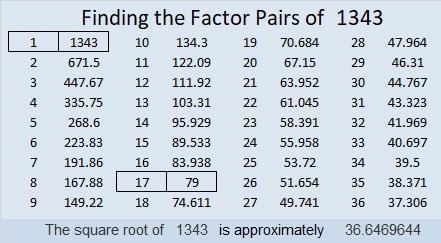1343 is the hypotenuse of a Pythagorean triple:
632-1185-1343 which is (8-15-17) times 79

Stetson.edu informs us that 1343 is 16 numbers away from the closest prime number, and it is the smallest number that can make that claim.

# 1334 and Level 2

You only need a few clues in the right places to figure out where all the factors from 1 to 12 belong in this mixed up multiplication table puzzle. You can use those clues to put the factors in the right places and solve this one!Print the puzzles or type the solution in this excel file: 12 factors 1333-1341

• 1334 is a composite number.
• Prime factorization: 1334 = 2 × 23 × 29
• 1334 has no exponents greater than 1 in its prime factorization, so √1334 cannot be simplified.
• The exponents in the prime factorization are 1, 1, and 1. Adding one to each exponent and multiplying we get (1 + 1)(1 + 1)(1 + 1) = 2 × 2 × 2 = 8. Therefore 1334 has exactly 8 factors.
• The factors of 1334 are outlined with their factor pairs in the graphic below.1334 is the hypotenuse of a Pythagorean triple:
920-966-1334 which is (20-21-29) times 46

It is also the leg in a couple of triples:
312-1334-1370 calculated from 29² – 23², 2(29)(23), 29² + 23²
1334-444888-444890 calculated from 2(667)(1). 667² – 1² , 667² + 1²

# 1322 Christmas Star

The first Christmas Star led the wise men to find the Baby Jesus.

This Christmas star can lead you to a better knowledge of all the facts in a basic 1 to 10 multiplication table.

Print the puzzles or type the solution in this excel file:10-factors-1321-1332

Now I’ll share a few facts about the number 1322:

• 1322 is a composite number.
• Prime factorization: 1322 = 2 × 661
• The exponents in the prime factorization are 1 and 1. Adding one to each and multiplying we get (1 + 1)(1 + 1) = 2 × 2 = 4. Therefore 1322 has exactly 4 factors.
• Factors of 1322: 1, 2, 661, 1322
• Factor pairs: 1322 = 1 × 1322 or 2 × 661
• 1322 has no square factors that allow its square root to be simplified. √1322 ≈ 36.35932

1322 is the sum of six consecutive prime numbers:
199 + 211 + 223 + 227 + 229 + 233 = 1322

1322 is the sum of two squares:
31² + 19² = 1322

1322 is the hypotenuse of a Pythagorean triple:
600-1178-1322 calculated from 31² – 19², 2(31)(19), 31² + 19²